• Verilog移位寄存器 HDLBits链接 前言 今天更新一节寄存器相关内容，其中涉及CRC校验的内容是用线性反馈移位寄存器搭建而成的。 题库 题目描述1： 构建一个4bit的移位寄存器(右移)，含异步复位、同步加载和使能 ...
Verilog移位寄存器
HDLBits链接

前言
今天更新一节寄存器相关内容，其中涉及CRC校验的内容是用线性反馈移位寄存器搭建而成的。

题库
题目描述1：
构建一个4bit的移位寄存器(右移)，含异步复位、同步加载和使能
Solution1：
module top_module(
input clk,
input areset,  // async active-high reset to zero
input ena,
input [3:0] data,
output reg [3:0] q);

always @(posedge clk or posedge areset)begin
if(areset)begin
q <= 4'b0;
end
q <= data;
end
else if(ena)begin
q <= {1'b0,q[3:1]};
end
else begin
q <= q;
end
end

endmodule

题目描述2：
Solution2：
module top_module(
input clk,
input [1:0] ena,
input [99:0] data,
output reg [99:0] q);

always @(posedge clk) begin
q <= data;
end
else begin
case (ena)
2'b01:q <= {q,q[99:1]};
2'b10:q <= {q[98:0],q};
default:q <= q;
endcase
end
end

endmodule

题目描述3：
建立一个64位算术移位寄存器，同步加载。移位器可以左右移位，并按数量选择1位或8位的移位。
Solution3：
module top_module(
input clk,
input ena,
input [1:0] amount,
input [63:0] data,
output reg [63:0] q);

always @(posedge clk)begin
q <= data;
end
else begin
if(ena)begin
case(amount)
2'b00: q <= {q[62:0],1'b0};
2'b01: q <= {q[55:0],8'b0};
2'b10: q <= {q,q[63:1]};
2'b11: q <= {{8{q}},q[63:8]};
endcase
end
else begin
q <= q;
end
end
end

endmodule

题目描述4：
构造线性移位寄存器，reset应当使LFSR归1。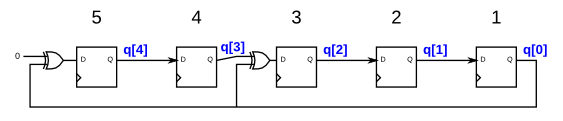Solution4：
module top_module(
input clk,
input reset,    // Active-high synchronous reset to 5'h1
output [4:0] q
);

always @(posedge clk)begin
if(reset)begin
q <= 5'h1;
end
else begin
q <= 1'b0 ^ q;
q <= q;
q <= q ^ q;
q <= q;
q <= q;
end
end

endmodule

题目描述5：
为这个序列电路编写Verilog代码。假设你要在DE1-SoC板上实现这个电路。将R输入连接到SW开关，将时钟连接到密钥，将L连接到密钥，将Q输出连接到红灯LEDR上。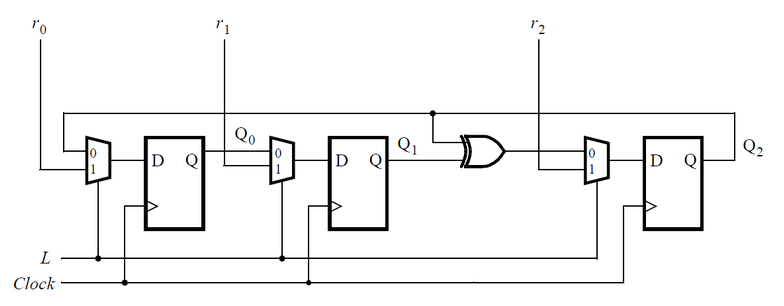Solution5：
module top_module (
input [2:0] SW,      // R
input [1:0] KEY,     // L and clk
output [2:0] LEDR);  // Q

wire clk;
assign clk = KEY;

always @(posedge clk)begin
if(KEY)begin
LEDR <= SW;
LEDR <= SW;
LEDR <= SW;
end
else begin
LEDR <= LEDR;
LEDR <= LEDR;
LEDR <= LEDR ^ LEDR;
end
end

endmodule

题目描述5：
构建一个32位的Galois LFSR，其taps位置为32、22、2和1。
Solution5：
module top_module(
input clk,
input reset,    // Active-high synchronous reset to 32'h1
output [31:0] q
);
integer i;
always @(posedge clk)begin
if(reset)begin
q <= 32'h1;
end
else begin
for(i=0;i<32;i++)begin
if((i==21)||(i==1)||(i==0))begin
q[i] <= q[i+1] ^ q;
end
else if(i==31)begin
q <= 1'b0 ^ q;
end
else begin
q[i] <= q[i+1];
end
end
end
end

endmodule

**题目描述6：**实现如下电路图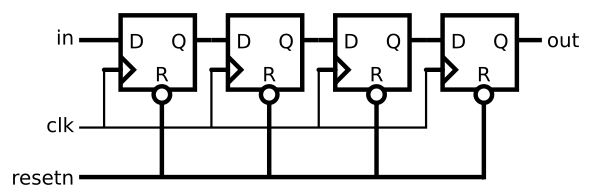Solution6：
module top_module (
input clk,
input resetn,   // synchronous reset
input in,
output out);

reg [3:0] tmp;
assign out = tmp;

always @(posedge clk)begin
if(!resetn)begin
tmp <= 4'h0;
end
else begin
tmp <= {tmp[3:1],in};
end
end

endmodule

**题目描述7：**实现如下电路图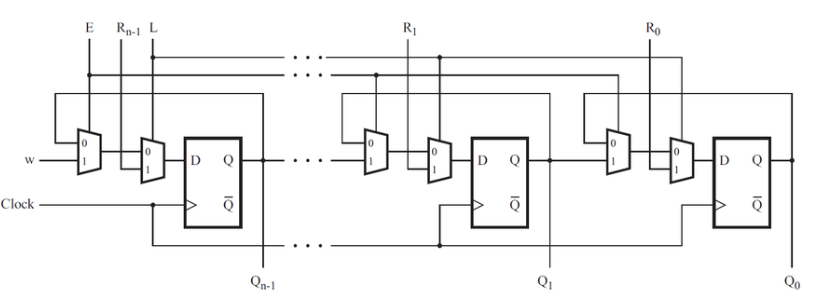Connect the R inputs to the SW switches,clk to KEY,E to KEY,L to KEY, andw to KEY.Connect the outputs to the red lights LEDR[3:0].
Solution7：
module top_module (
input [3:0] SW,
input [3:0] KEY,
output [3:0] LEDR
);

MUXDFF u1(.clk(KEY),
.w(KEY),
.R(SW),
.E(KEY),
.L(KEY),
.Q(LEDR));

MUXDFF u2(.clk(KEY),
.w(LEDR),
.R(SW),
.E(KEY),
.L(KEY),
.Q(LEDR));

MUXDFF u3(.clk(KEY),
.w(LEDR),
.R(SW),
.E(KEY),
.L(KEY),
.Q(LEDR));

MUXDFF u4(.clk(KEY),
.w(LEDR),
.R(SW),
.E(KEY),
.L(KEY),
.Q(LEDR));

endmodule

module MUXDFF (
input clk,
input w,R,E,L,
output Q
);
wire tmp;
assign tmp = E ? w : Q;
always @(posedge clk)begin
Q <= L? R : tmp;
end

endmodule

题目描述8：
在这个问题中，你将为一个8x1存储器设计一个电路，在这个电路中，写入到存储器是通过移位来完成的，而读取是“随机访问”，就像在一个典型的RAM中一样。然后您将使用该电路实现一个3输入逻辑功能。
首先，用8个d类型触发器创建一个8位移位寄存器。标记为Q到Q。移位寄存器输入称为S，输入Q (MSB先移位)。使能输入enable控制是否移位，扩展电路使其有3个额外的输入A,B,C和一个输出Z。电路的行为应该如下:当ABC为000时，Z=Q，当ABC为001时，Z=Q，以此类推。你的电路应该只包含8位移位寄存器和多路复用器。(这个电路称为3输入查找表(LUT))。
Solution8：
module top_module (
input clk,
input enable,
input S,
input A, B, C,
output Z );

reg [7:0] Q;
always @(posedge clk)begin
if(enable)begin
Q <= {Q[6:0],S};
end
else begin
Q <= Q;
end
end

assign Z = Q[{A,B,C}];

endmodule


小结
今天更新了部分移位寄存器部分的答案，注意最后一题用了一些技巧来简化代码书写，但实现时电路并无差异，体现了HDL中Describe的特性。之后一段时间还要忙比赛，有空再继续更，希望队伍比赛顺利。
展开全文• Verilog移位寄存器 HDLBits链接 前言 今天更新一节内容，该小节题目不多，共三道，但技巧性挺强。 题库 题目描述1：各单元的下一状态是此时当前单元相邻两位的异或。 在这个电路中，创建一个512单元系统(q(511:0))...
Verilog移位寄存器
HDLBits链接

前言
今天更新一节内容，该小节题目不多，共三道，但技巧性挺强。

题库
题目描述1：各单元的下一状态是此时当前单元相邻两位的异或。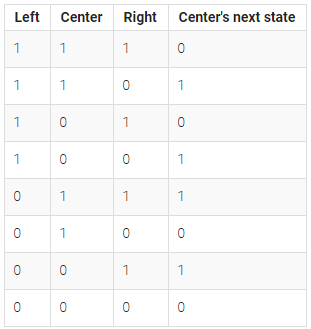Solution1：
module top_module(
input clk,
input [511:0] data,
output [511:0] q );

always @(posedge clk)begin
q <= data;
end
else begin
q <= {1'b0,q[511:1]}^{q[510:0],1'b0};
end
end

endmodule

题目描述2：
与题目一类似，状态转移条件发生变化，如下图所示：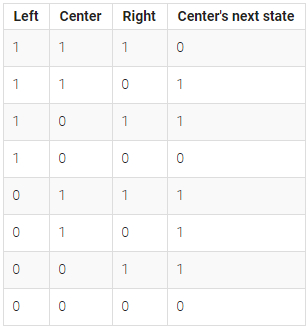Solution2：
module top_module(
input clk,
input [511:0] data,
output [511:0] q
);

always @(posedge clk)begin
q <= data;
end
else begin
q <= (~{1'b0,q[511:1]} & q) | (q & ~{q[510:0],1'b0}) | {~{1'b0,q[511:1]} & {q[510:0],1'b0}} | {~q & {q[510:0],1'b0}};
end
end

endmodule

tips：进行卡诺图化简后可看懂q状态转移的含义。
题目描述3：
一个中心点周围有8个邻居，如果周围的邻居中1的数目为0-1个，那么中心点变为0；如果周围邻居中1的数目为2个，那么中心点状态不变；如果周围邻居中1的数目为3个，中心点变为1；如果周围邻居中1的数目大于3个，中心点变为0。
我们可以将周围的8个邻居的值都加起来来判断周围邻居中1的个数，值得注意的是，这里我们在for中使用了阻塞赋值，因需要当前拍（本周期）得到结果在当前拍（本周期）就去判断。
建议大家做该题的时候花一个16*16的方阵，分析边界条件，达到事半功倍的效果。
生命游戏之父约翰·康威因感染新冠于2020年4月11日去世，享年83岁，在此表示哀悼。
Solution3：
module top_module(
input clk,
input [255:0] data,
output [255:0] q );

reg [3:0] count;
integer i;

always @(posedge clk)begin
q <= data;
end
else begin
for(i=0;i<256;i++)begin
if(i == 0)begin
count = q + q + q + q + q + q + q + q;
end
else if(i == 15)begin
count = q + q + q + q + q + q + q + q;
end
else if(i == 240)begin
count = q + q + q + q + q + q + q + q;
end
else if(i == 255)begin
count = q + q + q + q + q + q + q + q;
end
else if( i>0 && i<15)begin
count = q[239+i]+q[240+i]+q[241+i]+q[i-1]+q[i+1]+q[i+15]+q[i+16]+q[i+17];
end
else if(i>240 && i<255)begin
count = q[i-17]+q[i-16]+q[i-15]+q[i-1]+q[i+1]+q[i-239]+q[i-240]+q[i-241];
end
else if( i%16 == 0)begin
count = q[i-1]+q[i-16]+q[i-15]+q[i+15]+q[i+1]+q[i+31]+q[i+16]+q[i+17];
end
else if(i % 16 == 15)begin
count = q[i-17]+q[i-16]+q[i-31]+q[i-1]+q[i-15]+q[i+15]+q[i+16]+q[i+1];
end
else begin
count = q[i-17]+q[i-16]+q[i-15]+q[i-1]+q[i+1]+q[i+15]+q[i+16]+q[i+17];
end

case(count)
4'd2:q[i] <= q[i];
4'd3:q[i] <= 1'b1;
default:q[i] <= 1'b0;
endcase
end
end
end

endmodule


小结
本次练习主要难在第三题中的阻塞赋值，因为我们需在当前周期内算出count并对当前算出的count值进行判断，需用阻塞赋值。
希望自己以后遇到问题能够慢慢划分解决，徐图缓进
展开全文• Verilog写一个简单的多功能移位寄存器，并在移位寄存器的基础上进行修改，利用移位功能形成流水灯的效果 基本的功能如下： 寄存器示意图见下方虚拟面板图 有两种改进方式： 第一种是把最后一个功能改成循环右移，...
用Verilog写一个简单的多功能移位寄存器，并在移位寄存器的基础上进行修改，利用移位功能形成流水灯的效果 基本的功能如下：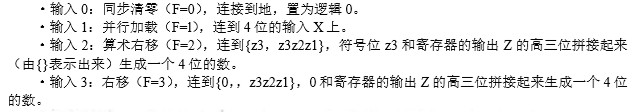寄存器示意图见下方虚拟面板图 有两种改进方式： 第一种是把最后一个功能改成循环右移，即此功能模式下，按一下时钟按键（代表时钟信号到来），高三位往右移一位，最低位移动到最高位。按一次移位一次，形成流水灯效果 第二种在第一种的基础上把按键时钟改成接入内部时钟，每隔一段时间时钟信号来临一次，无需手动操作，形成自动移位的流水灯效果
基本功能的移位寄存器的主要文件Virtual_Lab_Top.v中的代码：
//移位寄存器实验
default_nettype none
module Virtual_Lab_Top
(
input  wire  CLOCK,
input  wire  [4:0] BUTTON, //按键
input  wire [35:0] SWITCH, //开关
output wire [35:0] LED,    //指示灯
output wire  [3:0] HEX0,   //数码管
output reg   [3:0] Z
);

//输入端口赋值给内部信号
wire C    = BUTTON;
wire R    = BUTTON;
wire E    = SWITCH;
wire f1   = SWITCH;
wire f0   = SWITCH;
wire [3:0] X = SWITCH[3:0];

//实验逻辑
wire i;
wire [3:0] D;
assign i = f1&~f0&Z;
assign D = {4{~f1&f0}}&X|{4{f1&i}};

always @ (posedge C or posedge R)
begin
if(R)
Z<=0;
else if(C&E&~f1)
Z<=D;
else
Z<={i,Z[3:1]};
end

//内部信号赋值给输出端口（指示灯）观察
assign LED[3:0]  = Z[3:0];

//内部信号赋值给输出端口（数码管）观察
assign HEX0 = D;

endmodule

第一种改进后主要文件Virtual_Lab_Top.v中的代码：
//流水灯实验
default_nettype none
module Virtual_Lab_Top
(
input  wire  CLOCK,
input  wire  [4:0] BUTTON, //按键
input  wire [35:0] SWITCH, //开关
output wire [35:0] LED,    //指示灯
output reg   [3:0] Z
);

//输入端口赋值给内部信号
wire C    = BUTTON;
wire R    = BUTTON;
wire E    = SWITCH;
wire f1   = SWITCH;
wire f0   = SWITCH;
wire [3:0] X = SWITCH[3:0];

//实验逻辑
wire i;
assign i = f1&~f0&Z;

always @ (posedge C or posedge R)
begin
if(R)
Z<=0;
else if(C&E&~f1&~f0)
Z<={Z,Z[3:1]};
else if(C&E&~f1&f0)
Z<=X;
else
Z<={i,Z[3:1]};
end

//内部信号赋值给输出端口（指示灯）观察
assign LED[3:0]  = Z[3:0];

endmodule

第二种改进： 把第一种改进的代码中的 wire C = BUTTON; 这条语句删掉，然后把 always @ (posedge C or posedge R) 语句中的C改成CLOCK。我们要接入内部时钟，所以要先把按键时钟删掉。 加入分频器文件clock_divider.v，采用的是偶分频方法。一般板子的晶振频率太高，所以要将原有时钟频率分频降低，常见板子的晶振频率为50MHz，以这个频率为标准，在工程中的JuLabPocket_TOP.v文件中，将直接接入原频率的语句 wire CLOCK = CLOCK_50; 删去，然后在该文件合适处加入下列语句
//------------ 时钟 --------------------------------------------------------//
wire CLOCK_divided;
//使用时钟分频器模块产生1kHz的时钟。可以修改RATIO参数改变输出频率。
clock_divider #(.RATIO(10000000)) clk_inst(.clkin(CLOCK_50), .rst(1'b0), .clkout(CLOCK_divided));

wire CLOCK = CLOCK_divided;  //用分频后的CLOCK_divided作为时钟


这样调用了分频器给时钟信号变量接入分频后的频率，如果流水灯移位的速度还是太快，可以把参数RATIO调大，太慢就调小，建议直接在后面加一个0或减一个0或着减半
完整的工程文件我已经上传到CSDN了，可以去我的主页查看并下载完整的工程文件进行使用,压缩包中有三个文件夹，对应三个版本的完整工程文件，基础版本的是shifter文件夹，第一种改进的是led文件夹，第二种改进的是CLOCK & led文件夹，若CLOCK & led文件夹的工程文件不能在Quartus II中正常打开，则把文件夹名中的&符号删掉再打开即可 前两种版本的工程内包含文件如下图，第三种的多了一个clock_divider.v：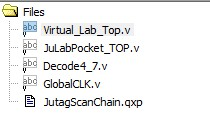WeLab虚拟面板设置图片：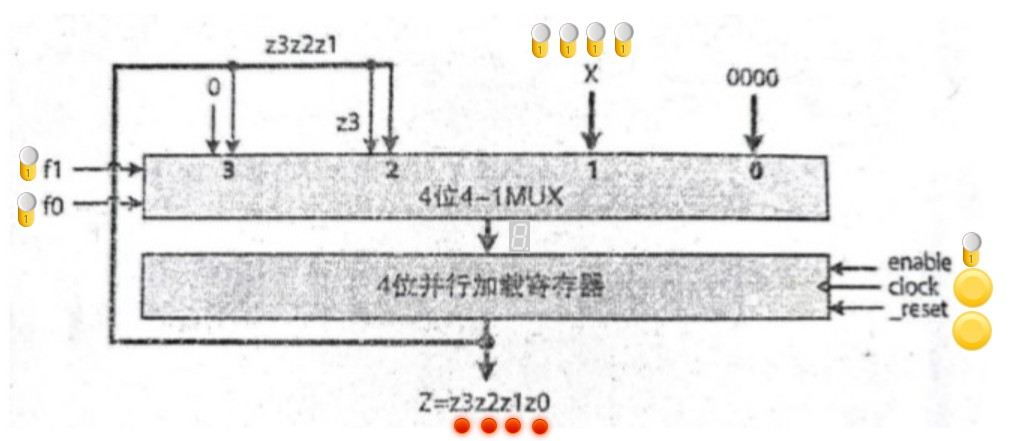以上为基础版本，第一种改进版本删去数码管，第二种改进版本删去clock时钟对应的按键 X输入数据，f1、f0是输入控制位F的高位和地位，数码管表示选择器的结果，enable开关置1则使能，置0则不使能，重置按键重置显示结果，按键时钟按下即代表时钟信号来临一次
注意：接入内部时钟的时候虚拟实验板由于软件的接收静态数据原因可能显示不出来流水灯的自动移位动态效果，但实际板子上会有效果显示出来，实际板子上分配的led灯真的会有流水灯的自动移位动态效果，如果是使用实际的实验板可以观察实验板
展开全文• 这个文件详细的描述了一位寄存器的实现过程还有testbench，主要是以word的形式存放的
• verilog 描述移位寄存器 并用multisim生成电路图 。
• 一些用Modelsim仿真的verilog源代码，包括计数器，移位寄存器等。
• verilog变长移位寄存器 普通的移位寄存器可以按如下设置，下面是一个一次移动一位的移位寄存器。 module shift_stationary( clk, dat, ctrl, shift_out ); input clk; input [15:0]dat; input [1:0]ctrl; ...
verilog变长移位寄存器
普通的移位寄存器可以按如下设置，下面是一个一次移动一位的移位寄存器。
module shift_stationary(

clk,
dat,
ctrl,
shift_out
);
input clk;
input [15:0]dat;
input [1:0]ctrl;
output reg [15:0] shift_out;

always@(posedge clk)
begin
case(ctrl)
2'b00:
begin
shift_out={dat[14:0],1'b0};
end
2'b01:
begin
shift_out={dat[14:0],dat};
end
2'b10:
begin
shift_out={dat,dat[15:1]};
end
2'b11:
begin
shift_out={1'b0,dat[15:1]};
end
endcase
end

endmodule

如何根据输入来决定移位寄存器要移动几位？采用变长的移位寄存器：
module shift
(
clk,
dat,
shift_n,
lshift_out
);
input clk;
input [15:0]dat;
input [4:0]shift_n;
output reg [15:0] lshift_out;

always@(posedge clk)
begin
lshift_out=dat<<shift_n;
end

endmodule

可以根据输入值确定移动几位。以上的代码可以综合成功。
展开全文• 目录 背景 ...环形移位寄存器（右移） Verilog HDL描述 测试代码 仿真波形图 ISE综合 背景 之所以单独把这个简单的东西拿出来，就是因为这个东西我可能要用到，不能眼高手低，以为简单就一...
• ## 移位寄存器专题（verilog HDL设计）

万次阅读 多人点赞 2018-05-19 21:09:11
移位寄存器简介 分类 4位右移位寄存器工作原理 1、16位右移位寄存器 2、16位左移寄存器 3、串行输入并行输出寄存器 4、并行输入串行输出移位寄存器 移位寄存器简介 移位寄存器内的数据可以在移位脉冲（时钟...并行输入串行输出
• 前言   之前老是想着学的快点，就直接编译了程序就下载在开发板上跑，后来发现这样不行，因为如果程序有问题，验证和纠错的时间成本太高了...移位寄存器程序代码： `timescale 1ns / 1ps /////////////////////////fpga
• 在数字电子产品中，移位寄存器是级联的触发器，其中一个触发器的输出引脚q连接到下一个触发器的数据输入引脚（d）。 因为所有触发器都在同一时钟上工作，所以存储在移位寄存器中的位阵列将移位一个位置。
• 线性反馈移位寄存器（linear feedback shift register, LFSR）是指，给定前一状态的输出，将该输出的线性函数再用作输入的移位寄存器。异或运算是最常见的单比特线性函数：对寄存器的某些位进行异或操作后作为输入，...fpga
• Verilog-LFSR线性反馈移位寄存器1 LFSR 是什么2 几道题目2.1 题目1：5-bit LFSR2.2 题目2：3-bit LFSR2.3 题目3：32-bit LFSR 1 LFSR 是什么 在HDLBits网站做到了几道关于LFSR，Liner Feedback Shift Register线性......